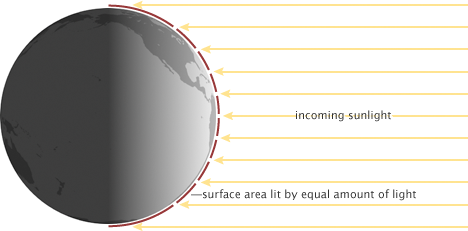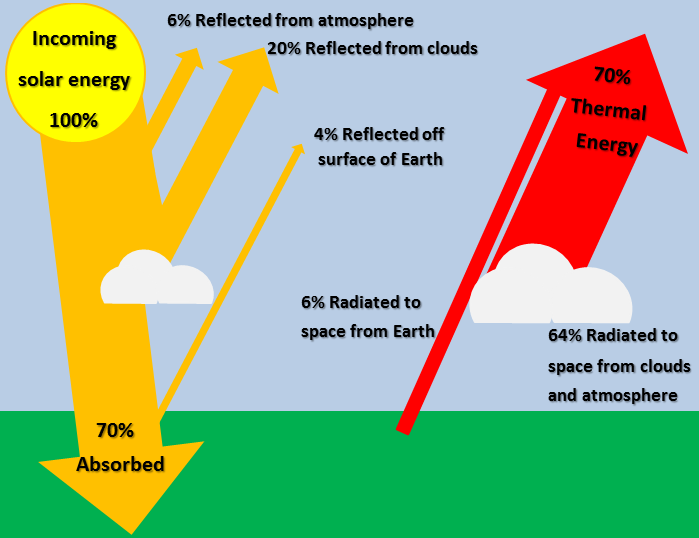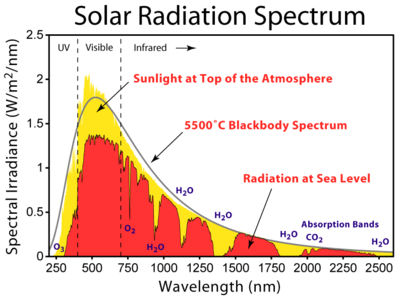# Solar energy to the EarthFigure 1. The Sun is the major source of energy and vital to life on Earth, but much of its light is reflected. Solar energy acts as a primary energy flow that can be harnessed.

Almost all of the Earth's energy input comes from the sun. Not all of the sunlight that strikes the top of the atmosphere is converted into energy at the surface of the Earth. The Solar energy to the Earth refers to this energy that hits the surface of the Earth itself. The amount of energy that reaches the the Earth provides a useful understanding of the energy for the Earth as a system. This energy goes towards weather, keeping the temperature of the Earth at a suitable level for life, and powers the entire biosphere. Additionally, this solar energy can be used for solar power either with solar thermal power plants or photovoltaic cells.

## Calculating Solar Energy to Surface

### Energy from Sun to Earth

The Sun is generally considered to produce a constant amount of power (although there are small variances in the output energy depending on sunspot cycles) with a surface intensity of , expressed in units of power per unit area. As the Sun's rays spread into space this radiation becomes less and less intense as an inverse square law. The average radiation intensity that hits the edge of the Earth's atmosphere is known as the solar constant, or . Although this value is called a constant it varies by about 7% between January 4th (perihelion), when the Earth is closest to the sun, and July 4th (aphelion), when the Earth is furthest away. Therefore a yearly average is used and is determined to be . To determine this value from solar flux, the distance from the Earth to the Sun is used. As well, the total solar flux - not solar flux per unit area - must be determined. Then the total solar flux from the Sun is divided by the surface area of a sphere that has a radius equal to the distance from the Earth to the Sun. This accounts for the "spreading" of the solar energy. The expression to determine this value is:

To determine the average amount of solar energy that reaches the Earth, we must consider what the Earth "looks like" to the Sun. When looking at Earth from the Sun, only one half of the Earth can be seen. Thus to make an appropriate estimate of the average amount of solar energy over the entire surface area of the Earth the value for must be divided by 2.Figure 2. How the intensity of sunlight on Earth varies with lattitude.

In addition to this, the total solar irradiance is the maximum power delivered to a surface assuming that the path of incoming light is perpendicular to that surface. Due to the fact that the Earth is a sphere, only places near the equator come close to this perpendicular angle. At all other locations on the Earth, incoming sunlight is at some angle. With this decreasing angle, the average solar irradiance decreases as well. To account for this, the solar irradiance level is again halved, leaving a final average solar irradiance of approximately .

This value, which represents the average solar irradiance hitting Earth's outer atmosphere, does not yet account for losses due to reflection and absorption, which is discussed in the following section.

### Energy Absorbed by the Earth

Not all of the solar energy that reaches the Earth's atmosphere is absorbed by the Earth. This is due to something known as the Earth's energy budget. This budget accounts for the fact that some of the energy incident on the outer atmosphere of the planet is immediately reflected back into space.Figure 3. Percentage of incoming radiation from the Sun that is reflected and absorbed by the surface of the Earth, the atmosphere, and clouds.

Due to reflection by the atmosphere, clouds, and Earth's surface we can approximate that 70% of solar energy incident on the edge of the Earth's atmosphere is actually absorbed by the Earth. Taking this into account, the actual average amount of solar energy absorbed by the Earth amounts to:

### Energy to the Surface

Energy that is absorbed by the Earth is not the same as the energy incident on the Earth's surface. On a perfectly clear or cloudless day, when the Sun is directly overhead (or at the "zenith"), solar irradiation is still reduced due to absorption (16%) and reflection (6%) by particles in Earth's atmosphere. These particles include carbon dioxide (CO2), Oxygen (O2), Ozone (O3) and water vapor (H2O).Figure 4. Solar radiation spectrum for direct light at both the top of the Earth's atmosphere and at sea level.

This 22% reduction of solar irradiation will be higher on average because the Sun is not always at the zenith. To standardize this measurement, a unit called Air Mass is used to define the solar spectrum that is incident at various altitudes and conditions on Earth. Air Mass 0, or AM0 spectrum is the solar radiation outside the atmosphere and represents a power density of . AM1.5 is regarded as "normal" air mass and represents a power density of .

The quantity of is defined as a unit of radiation, called "one sun", and is commonly used as a standard to test and rate photovoltaic cells and solar panels. This means that a solar panel rated at 250 watts will output this rated power when exposed to a solar power density of . Although this amount of energy is quite significant, it does not mean that solar energy can easily provide all of our primary energy. Problems with solar energy include cloudy days and the lack of a reasonable way to store "excess" energy collected on sunny days. As well, this assumes that solar panels are 100% efficient at converting solar energy into electricity or another usable form of energy, which is not the case. Although solar power is one important type of renewable energy source, it is important to investigate the benefits and drawbacks of this type of energy.